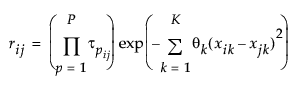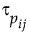Publication date: 05/24/2021

##Models with Categorical Predictors

If the Gaussian Process model includes categorical predictors, the Gaussian correlation structure is used for the correlation structure. The elements of the R matrix are defined as follows:where

K = # of continuous predictors

P = # of categorical predictors

θk = theta parameter for the kth continuous predictor

xik = the value of the kth continuous predictor for subject i

xjk = the value of the kth continuous predictor for subject j= the correlation between the observed level of predictor p for subject i and the observed level of predictor p for subject j

There is a τ parameter for each combination of levels of a categorical variable, where τij corresponds to the unique combination formed by the observed levels of subject i and subject j. Thus, the covariance element, rij, depends on the combination of levels of the categorical predictors obtained from the ith and jth observations. See Qian et al. (2012).